# MA.912.AR.1.4Export Print
Divide a polynomial expression by a monomial expression with rational number coefficients.

### Clarifications

Clarification 1: Within the Algebra 1 course, polynomial expressions are limited to 3 or fewer terms.
General Information
Subject Area: Mathematics (B.E.S.T.)
Strand: Algebraic Reasoning
Status: State Board Approved

## Benchmark Instructional Guide

• Monomial
• Polynomial

### Vertical Alignment

Previous Benchmarks

Next Benchmarks

### Purpose and Instructional Strategies

In middles grades, students added, subtracted and multiplied linear expressions. In Algebra I, students perform operations on polynomials limited to 3 or fewer terms and divide polynomials by a monomial. In later courses, students will perform operations on all polynomials.
• Instruction includes the connection to addition, subtraction and multiplication of polynomials to develop the understanding of closure, and the connection to properties of exponents.
• Instruction includes proper vocabulary and terminology, keeping in mind that when dividing, the word “cancel” can become a misconception for students. A number or an expression divided by itself is equivalent to 1 and does not disappear (see Appendix D).
• Instruction includes the use of manipulatives, like algebra tiles, and various strategies, like the area model, properties of exponents and decomposing fractions.
• Decomposing fractions
•  The expression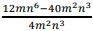can be written as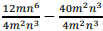. Students can then   perform the division with  each fraction to determine the difference.

### Common Misconceptions or Errors

• Students may not understand the meaning of closure (although not directly discussed in this benchmark, polynomials are not closed under division).
• Students may not understand like terms.

### Strategies to Support Tiered Instruction

• Teacher provides examples showing that polynomials are closed under the operations of addition, subtraction and multiplication, but not under division.
• For example, when subtracting or multiplying polynomials (as shown below), the result is always a polynomial.
7$x$ +5−(3$x$ + 8) = 4$x$ − 3
(7$x$ + 5)(3$x$ + 8) = 21$x$2 + 71$x$ + 40
• For example, when dividing polynomials (as shown below), the result may or may not be a polynomial.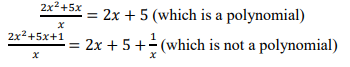Instructional Task 1 (MTR.3.1, MTR.4.1, MTR.5.1)
• Part A. Determine the quotient of ($\frac{\text{1}}{\text{3}}$$a$4 − 3$a$3 + $\frac{\text{1}}{\text{2}}$$a$2) and 3$a$3
• Part B. Discuss with your partner the strategy used. How do your quotients compare to one another?

• Part A. Determine the quotient of $x$ + $x$and $x$-1
• Part C. What happens when you divide $x$ + $x$2 by the expression $x$$\frac{\text{1}}{\text{2}}$ (which is not a monomial)?

### Instructional Items

Instructional Item 1
• What is the quotient of the expression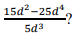Instructional Item 2
• What is the quotient of the expression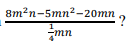Instructional Item 3
•  What is the quotient of the expression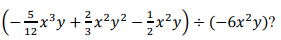*The strategies, tasks and items included in the B1G-M are examples and should not be considered comprehensive.

## Related Courses

This benchmark is part of these courses.
1200310: Algebra 1 (Specifically in versions: 2014 - 2015, 2015 - 2022, 2022 and beyond (current))
1200320: Algebra 1 Honors (Specifically in versions: 2014 - 2015, 2015 - 2022, 2022 and beyond (current))
1200380: Algebra 1-B (Specifically in versions: 2014 - 2015, 2015 - 2022, 2022 and beyond (current))
1200400: Foundational Skills in Mathematics 9-12 (Specifically in versions: 2014 - 2015, 2015 - 2022, 2022 and beyond (current))
7912090: Access Algebra 1B (Specifically in versions: 2014 - 2015, 2015 - 2018, 2018 - 2019, 2019 - 2022, 2022 and beyond (current))
1200315: Algebra 1 for Credit Recovery (Specifically in versions: 2014 - 2015, 2015 - 2022, 2022 and beyond (current))
1200385: Algebra 1-B for Credit Recovery (Specifically in versions: 2014 - 2015, 2015 - 2022, 2022 and beyond (current))
7912075: Access Algebra 1 (Specifically in versions: 2014 - 2015, 2015 - 2018, 2018 - 2019, 2019 - 2022, 2022 and beyond (current))

## Related Access Points

Alternate version of this benchmark for students with significant cognitive disabilities.
MA.912.AR.1.AP.4: Divide a polynomial expression by a monomial expression with integer coefficients.

## Related Resources

Vetted resources educators can use to teach the concepts and skills in this benchmark.

## Student Resources

Vetted resources students can use to learn the concepts and skills in this benchmark.

## Parent Resources

Vetted resources caregivers can use to help students learn the concepts and skills in this benchmark.